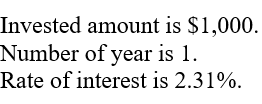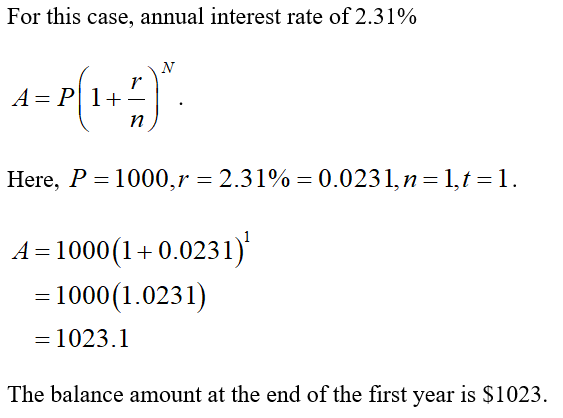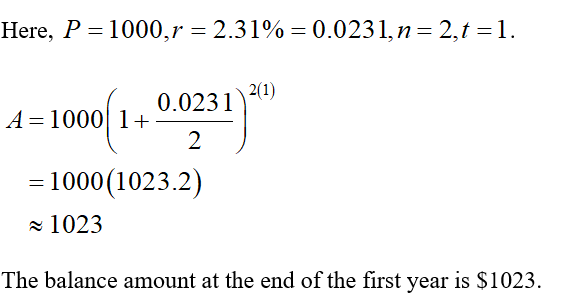# 2. You invest \$1, 000 in the beginning of a year and make no further deposits or withdrawals to and from this account. Find the balance at the end of the first year in each of the following cases:(a) the interest rate is an annual interest rate of 2.31%(b) the interest rate is a semi-annual interest rate of 2.31%(c) the interest rate is a quarterly interest rate of 2.31%.(d) Are the three interest rates above equivalent? Explain your answer.Which interest rate would you choose and why?

Question
10 views

2. You invest \$1, 000 in the beginning of a year and make no further deposits or withdrawals to and from this account. Find the balance at the end of the first year in each of the following cases:

(a) the interest rate is an annual interest rate of 2.31%

(b) the interest rate is a semi-annual interest rate of 2.31%

(c) the interest rate is a quarterly interest rate of 2.31%.

Which interest rate would you choose and why?

check_circle

Given Information:(a) Find the total amount at the end of first year.(b) Find the balance amount with interest rate is a semi-annual interest rate of 2.31%....

### Want to see the full answer?

See Solution

#### Want to see this answer and more?

Solutions are written by subject experts who are available 24/7. Questions are typically answered within 1 hour.*

See Solution
*Response times may vary by subject and question.
Tagged in